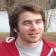# PHP: Basic Statistics Class

By Haktan Suren, PhD
In Blog
Oct 3rd, 2012
6860 Views

Recently, I used this PHP class in one of my projects. It is very useful, well-prepared and works great except one minor problem that I noticed. Standard deviation is miscalculated when you call the corresponding function.

When I checked the code, I realized it is due to the structure of the array causing the problem and make the output different than the STDEV function in Microsoft Excel.

I present the very quick fix of it for those who are also using this function.

```\$mean = \$this->mean;
\$XminA = array();
foreach(\$this->scores as \$key => \$val) {
(\$sqr == true) ? \$XminA[\$val] = round(pow((\$val - \$mean),2),\$this->round) : \$XminA[\$val] = round((\$val - \$mean),\$this->round);
}
if(\$sqr == true) {
```
```\$mean = \$this->mean;
\$XminA = array();
foreach(\$this->scores as \$key => \$val) {
(\$sqr == true) ? \$XminA[\$key] = round(pow((\$val - \$mean),2),\$this->round) : \$XminA[\$key] = round((\$val - \$mean),\$this->round);
}
if(\$sqr == true) {
```

Soon after I wrote this article, I realized the developer (Nathan Poultney) released the new version of the class.
Thanks for the update!Wrap your code in `<code class="{language}"></code>` tags to embed!